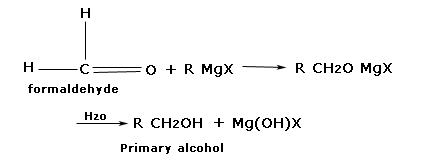Notes on General method of preparation of Alcohol | Grade 12 > Chemistry > Alcohols and Phenols | KULLABS.COM

Notes, Exercises, Videos, Tests and Things to Remember on General method of preparation of Alcohol

Please scroll down to get to the study materials.

• Note
• Things to remember

### 1. From haloalkane.

Haloalkane reacts with aueous alkali or moist AgO to give corresponding alcohols.

$$\underbrace{R-H}_{halo\,Alkane}\xrightarrow{aq.alkali\,or\,moist\,Ag_2O}\underbrace{R-OH}_{Alcohol}$$

Example.

$$\underbrace{CH_3CH_2Cl}_{Chloro\,ethane}\xrightarrow{aq.KOH}\underbrace{CH_3CH_2OH}_{Ethanol}+KCl$$

$$\underbrace{CH_3-CH-BR-CH_3}_{2-bromo\,propane}+Ag_2O(Moist)→\underbrace{CH_3-CH-OH-CH_3}_{2-propanol}+2AgBr$$

#### 2. From carbonyl compounds.

Carbonyl compounds C=O like aldehyde and ketones as well as other organic compounds such as carboxylic acid, esters, anhydrides are reduced to alcohols by the action of LiAlH4, NaBH4, or H2/Ni

$$\underbrace{C=O}_{Carbonyl\,Compound}+2[H]\xrightarrow{reducing\,agent}\underbrace{CH-OH}_{Alcohol}$$

Example.

$$\underbrace{CH_3-COH}_{Ethanal\,or\,Acetaldehyde)}+2[H]\xrightarrow{LiAlH_4}\underbrace{CH_3CH_2OH}_{Ethanol}$$

$$\underbrace{CH_3-CO-CH_3}_{Propanone\,or\,Acetone}+2[H]\xrightarrow{H_2\,Ni}\underbrace{CH_3-CH-OH-CH_3}_{2-Propanol}$$

$$\underbrace{CH_3-COOH}_{Ethanoic\,acid\,or\,Acetic\,acid}+H_2\xrightarrow{Ni}\underbrace{CH_3-CH_2-OH}_{Ethanol}+H_2O$$

$$\underbrace{CH_3-COOC_2H_5}_{Ethyl\,ethanoate\,or\,Ethyl\,acetate}\xrightarrow{LiAlH_4}\underbrace{2CH_3-CH_2OH}_{Ethanol}$$

3. From Grignards reagent.

Aldehydes and ketones from addition product with Grignard reagent. These addition products of hydrolysis in presence of an acid give alcohols. All three types of alcohol )10, 20, and 30) can be prepared by this method.

Preparation of primary alcohol.

Formaldehyde produces addition products with Grignard reagent which on hydrolysis from alcoholExample2 preparation of secondary alcohol.

All other aldehydes except formaldehyde produce secondary alcohol when treated with Grignard reagent followed by hydrolysis.Example.

3. Preparation of Tertiary alcohol.

Example.

4. From primary amines.

Primary amines react with nitrous acid to give corresponding alcohol.

$$\underbrace{R-NH_2}_{Primary\,amine}+HO-NO→\underbrace{R-OH}_{Alcohol}+N_2+H_2O$$

Example.

$$\underbrace{CH_3CH_2NH_2}_{Ethanamine}+HO-NO→\underbrace{CH_3-CH_2-OH}_{Ethanol}+N_2+H_2O$$

5. By hydrolysis of ester.

Esters can be hydrolyzed both in acidic and basic medium to give alcohol and carboxylic acid.

$$\underbrace{R-C=O-OR'}_{Ester}+H-OH\xrightarrow{H^+\,or\,OH^-}\underbrace{R-C=O-OH}_{Carboxylic\,acid}+\underbrace{R'-OH}_{Alcohol}$$

Reference.

Bahl, B S, Bahl, and Arun. Advanced Organic chemistry. S. Chand and company Ltd., n.d.

Sthapit, M K, R R Pradhananga, and K B Bajracharya. Foundations of chemistry. Taleju Prakashan, n.d.

Tewari, K S, S N Mehrotra, and N K Vishnoi. A textbook of organic chemistry. Vikash publishing House Pvt. ltd., n.d.

Verma, N K and S K Khanna. Compressive chemistry. 8th edition. Laxmi publications P. Ltd., 1999.

1. Haloalkane reacts with aqueous alkali or moist AgO to give corresponding alcohols.

$$\underbrace{R-H}_{halo\,Alkane}\xrightarrow{aq.alkali\,or\,moist\,Ag_2O}\underbrace{R-OH}_{Alcohol}$$

2. Carbonyl compounds C=O like aldehyde and ketones as well as other organic compounds such as carboxylic acid, esters, anhydrides are reduced to alcohols by the action of LiAlH4, NaBH4, or H2/Ni

3. $$\underbrace{C=O}_{Carbonyl\,Compound}+2[H]\xrightarrow{reducing\,agent}\underbrace{CH-OH}_{Alcohol}$$

.